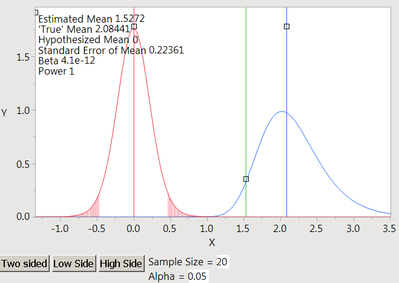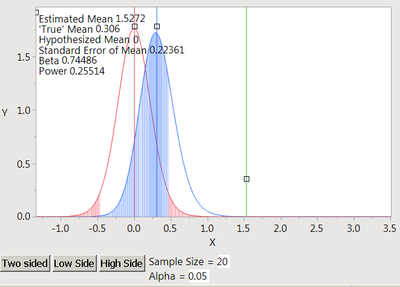Our World Statistics Day conversations have been a great reminder of how much statistics can inform our lives. Do you have an example of how statistics has made a difference in your life? Share your story with the Community!
Choose Language Hide Translation Bar

## Could someone explain to me the "One Sample Mean", "Power for Testing Mean " Animation Script?

Hello,

I was looking at some of the different teaching scripts and I noticed this one found in the "One Sample Mean", Animation Script "Power for Testing Mean".

I was confused on the following:

1. What is the 'green vertical bar' titled "Estimated Mean" do and what does this represent?

I tried moving the bar back and forth and it does not appear to change any of the calculated values (Power, std. error of mean, beta, etc..).

2. Am I correct in understanding that the " 'True Mean' " (blue distribution) is the average determined by the sample or is that what the "Estimated Mean" supposed to be?

3. Why is the distribution of the " 'True Mean' " changing as it gets further or closer to the "Hypothesized Mean"?

4. Is the "Hypothesized Mean" (red distribution) distribution a normal distribution?

5. Is the " 'True mean' " distribution a non-central F distribution?

I took snapshots of the script below.Thank you,
Nate

Highlighted

## Re: Could someone explain to me the "One Sample Mean", "Power for Testing Mean " Animation Script?

1. The green vertical line represents the sample average estimated from the data. "True" mean is initially set to this value.

2. Correct.

3. The distribution of the test statistic (i.e., one-sample t test) under the 'True" mean has a non-central t-distribution. When you move the "True" mean close to or away from the hypothesized mean, the probablity density function changes. The following figure shows the non-central t-distribution with different values of non-central parameter μ, and ν degree of freedom Noncentral t-distribution - Wikipedia, the free encyclopedia4. The red represents t-distribution.

5. The blue represents non-central t-distribution.

Article Labels

There are no labels assigned to this post.